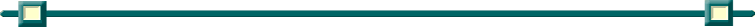The graphing calculator can be very useful for "checking" your
 1. Determine numerical equivalents. The calculator can be easily used to solve multiple choice style problems where numerical equivalents are needed.  Find a decimal answer to the problem and then check each answer to see which answer matches your decimal. The expression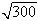is equivalent to      (1)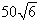(2)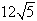(3)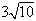(4)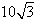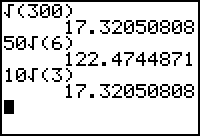Hint:  Get in the habit of CLOSING parentheses.  This habit will be most beneficial when working with longer problems.
 2. Be careful when comparing inequalities. Calculators (and computers) do not evaluate "sandwich" expressions written in the form 3 < x < 10 as you might expect.  They will only evaluate the first condition (3 < x).  To evaluate the entire expression, you will need to "tell" the calculator to also use the second expression by using the word "and".  Find "and" in the CATALOG or under the TEST menu → LOGIC.                    (3 < x < 10  must be entered as  3 < x  and  x < 10) If the relationship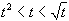is true, then t could be        (1)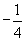(2) 0       (3)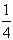(4) 4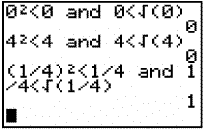The numerical choices have been substituted into the inequality expression. If the answer is 0, the expression is FALSE. If the answer is 1, the expression is TRUE. This is called a Boolean test.